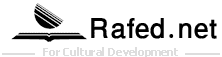## Possibilities and Probabilities

By : Ali R. Amir-Moez

Art by : Brenda Pepper

How many times have you heard someone say, "It's possible but not probable"? They mean that something could happen but probably wouldn't.

But what does it mean to say that something is probable or not probable? To get an idea, let's start with a simple experiment.

If you toss up a single coin, there are two possibilities for how it will land: heads or tails. The chance of getting heads is one out of two. Another way to say that is, the probability of getting heads is one-half. Or you can use percentages and say the probability of getting heads is fifty percent. Sometimes people say there is a fifty-fifty chance of getting heads.

Those are four different ways of saying the same thing. But a mathematician's way to say it is: The probability of getting heads is 1/2 ("one-half"). The probability of getting tails is also 1/2.

Of course, this idea does not predict exactly how the coin will land. If you do this experiment, then you may get more heads at first, or more tails. But as you toss the coin again and again, you will see that the total numbers give heads in about half the tosses and tails in about the other half. A probability predicts about how many events will happen, not exactly how many.

To try a more interesting coin-flipping experiment, use two different kinds of coins, such as a dime and a nickel. Count all possible ways they may land:
2. The dime is heads and the nickel is tails.
3. The dime is tails and the nickel is heads.
4. Both coins are tails.
So there are four, and only four, possibilities. The probability of each one is 1/4.

Try and See
Suppose you have a bowl of small squares cut from colored paper, such as construction paper. In this experiment, instead of tossing a coin, you will pick out squares without looking at them. If half of the squares are blue and half are yellow, what is the probability of your picking out a blue square? (Answer A, below.)

If the bowl contains 100 squares (25 each of blue, yellow, black, and white), what is the probability of getting a blue one? (Answer B, below.)

Using a chart like the one shown, record the results of taking a square out of such a bowl (and then mixing it back in) 40 times. Did you get about 10 blue ones? If you had a giant bowl of 10,000 squares and drew out 1,000, you would be even more likely to get 1 blue one for every 4 squares picked out.

Now that you've thought about these possibilities and probabilities, imagine you have 10,000 squares in a giant jar. All of the squares are yellow except one, which is blue. Without looking into the jar, would you be able to pick out the blue square on the first try?

Well, it's possible but not very probable.

Comment description

### Latest Post

• 2021-12-19 04:53:52 229
• 2021-12-12 06:26:45 307
• 2021-12-12 05:43:43 394

### Most Reviews2021/12/192021/12/122021/12/04